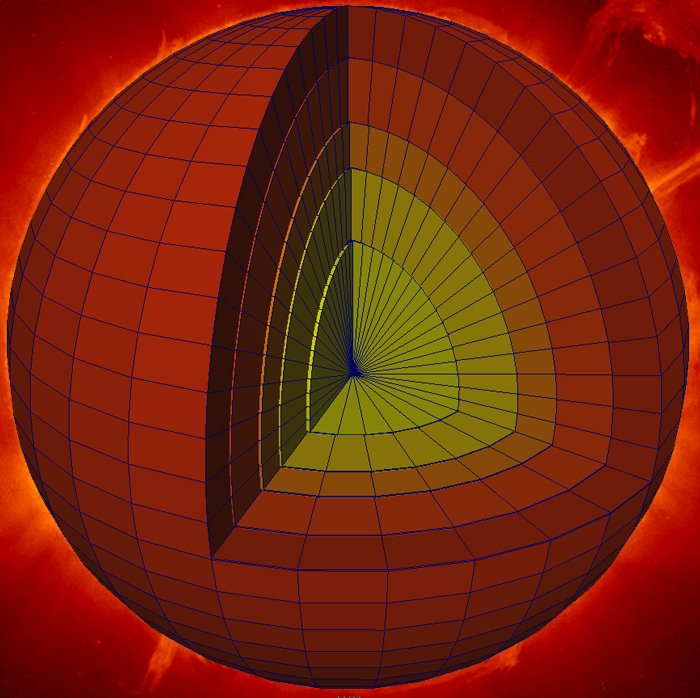### Members#### Matteo Bugli

PhD Student

Ninoy Rahman (Doktorand)

Kollegen, die häufig zu Besuch kommen:

Thomas W. Baumgarte (Bowdoin College, Maine, USA)
Martin Obergaulinger (Univ. Valencia, Spain)
Victor Utrobin (ITEP, Moscow, Russia)
Annop Wongwathanarat (RIKEN, Tokyo, Japan)

Derzeitige Besucher:

### Recent research highlights#### How a supernova obtains its shape

MPA researchers managed for the first time to reproduce the asymmetries and fast-moving iron clumps of observed supernovae by complex computer simulations in all three dimensions. [more]

# Computational Astrophysics

In astrophysics one is in the extraordinary situation, that the objects of interest (stars, galaxies, etc) are not accessible to any kind of manipulation. No experiments can be performed, and observations of a particular one-time event cannot be repeated.  The situation is further complicated because (i) the physical processes giving rise to the astrophysical phenomenon may occur deep inside the observed object, providing only indirect evidence of these processes, (ii) the processes may involve extreme conditions which are experimentally inaccessible in the laboratory, and (iii) the processes one tries to understand often occur on time scales long compared to the human life span, i.e., one is stuck with a snapshot of the phenomena.Zoom Image
An image of the sun (in He II 304 Å) taken on September 14, 1999 by the Extreme ultraviolet    Imaging Telescope (EIT) aboard the Solar and Heliospheric Observatory (SOHO). [less]Zoom Image
One-dimensional discretization of the sun (into a set of radial shells)Zoom Image
Three-dimensional discretization of the sun (into a set of blocks)

To proceed one constructs a theoretical model which incorporates all the physical processes thought to be of importance for the astrophysical phenomenon. The model, if of any value at all, should make some definite predictions about the properties or behavior of the object or phenomenon. This process requires simulation, because elaborated models typically consist of a set of nonlinear evolutionary equations. If the matter of astrophysical objects can be approximated as a gas or fluid, which is often the case, its evolution is governed by a set of conservation laws for mass, momentum, and energy. These hydrodynamic equations constitute a set of coupled nonlinear first-order (in time) partial differential equations. One way of solving this set of equations is to discretize the equations in time and space, whereby the partial differential equations are transformed into a set of coupled nonlinear algebraic equations, which can be solved on a computer with appropriate numerical techniques. As discretization introduces unavoidable errors, it is crucial to use discretization schemes, which minimize the errors.

Guided by the conservation properties underlying the hydrodynamic equations applied mathematicians and physicists have been able to develop accurate and stable high-resolution finite volume schemes. In these schemes the computational domain is discretized into a finite number of cells (typically 100 to 1000 per spatial dimension), and the continous hydrodynamic variables are approximated by cell averages. Time is discretized too, the evolution being approximated by a series of finite time steps, typically 1000 to 100000 per simulation.

Besides finite volume schemes, another completely different method is widely used in astrophysics for integrating the hydrodynamic equations. This method is Smoothed Particle Hydrodynamics, or SPH for short.  In SPH the fluid or gas is described by a finite set of "particles", which move with the flow.

## Funding sourcesEXC 153: Origin and Structure of the Universe - The Cluster of Excellence for Fundamental PhysicsGo to Editor View